欢迎访问企业培训网！ 免费注册 | 会员登陆 | 发布培训需求 | 下载培训APP | 将本站设为首页 | 加入收藏夹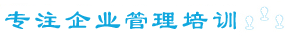培训课程 企业内训 商务考察 高校研修 培训讲师 管理学堂 培训专题
 精品培训课程推荐 ・高级文秘职业化素质训练班(深圳-上海-北京)  ・中层经理人管理技能提升研修班(上海-深圳)  ・张守春：3E薪酬体系设计与薪酬管理实战技巧  ・关键绩效指标与平衡计分卡(KPI/BSC)实战演练  ・应用Project软件进行项目管理实战演练培训班  ・现代企业档案管理全景实战(广州-上海-深圳)  ・EXCEL和PPT在管理中的高级运用(北京-上海)  ・清华大学系列研修班    北京大学系列研修班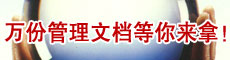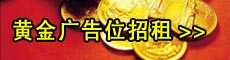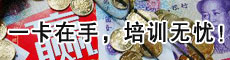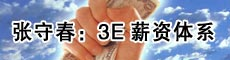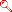长度
 1 millimetre 毫米= 0.03937 inch英寸 1 centimetre 厘米= 10 mm毫米=0.3937 inch英寸 1 decimetre 分米=10 cm厘米=3.937 inches英寸 1 metre 米=10 dm分米=1.0936 yards码=3.2808 feet英尺 1 kilometre 千米=1000 m米=0.6214 mile英里 1 mile marin 海里=1852 m米=1.1500 miles英里面积
 1 square centimetre平方厘米=0.155 sq.inch 平方英寸 1 square metre平方米=1.196 sq.yards 平方码 1 are 公亩=100 square metres 平方米=119.6 sq.yards 平方码 1 hectare 公顷=100 ares 公亩=2.471 acres 英亩 1 square kilometre 平方公里=0.386 sq.mile 平方英里体积
 1 cubic centimetre 立方厘米=0.061 cu.inch 立方英寸 1 cubic metre 立方米=1.308 cu.yards 立方码容积
 1 millilitre 毫升=0.002 British pint 英制品脱 1 centilitre 厘升=10 ml. 毫升=0.018 pint 品脱 1 decilitre 分升=10 cl. 厘升=0.176 pint 品脱 1 litre 升=10 dl. 分升=1.76 pints 品脱 1 decalitre 十升=10 l. 升=2.20 gallons 加伦 1 hectolitre 百升=100 l. 升=2.75 bushels 蒲式耳 1 kilolitre 千升=1000 l. 升=3.44 quarters 八蒲式耳重量
 1 milligram 毫克=0.015 grain 谷 1 centigram 厘克=10 mg. 毫克=0.154 grain 谷 1 decigram 分克=10 cg. 厘克=1.543 grains 谷 1 gram 克=10 dg. 分克=15.43 grains 谷 1 decagram 十克=10 g. 克=5.64 drams 打兰 1 hectogram 百克=100 g. 克=3.527 ounces 盎司 1 kilogram 千克=1000 g. 克=2.205 pounds 磅 1 ton (metric ton) 吨,公吨=1000 kg. 千克=0.984 (long) ton 英吨长度
 1 inch 英寸=25.4 millimetres 毫米 1 foot 英尺=12 inches 英寸=0.3048 metre 米 1 yard 码=3 feet 英尺=0.9144 metre 米 1 (statute) mile 英里=1760 yards 码=1.609 kilometres 千米 1 nautical mile 海里=1852 m. 米面积
 1 square inch 平方英寸=6.45 sq.centimetres 平方厘米 1 square foot 平方英尺=144 sq.in.平方英寸=9.29 平方分米 1 square yard 平方码=9 sq.ft. 平方英尺=0.836 sq.metre 平方米 1 acre 英亩=4840 sq.yd.平方码=0.405 hectare 公顷 1 square mile 平方英里=640 acres 英亩=259 hectares 公顷体积
 1 cubic inch 立方英寸=16.4 cu.centimetres 立方厘米 1 cubic foot 立方英尺=1728 cu.in. 立方英寸=0.0283 cu.metre 立方米 1 cubic yard 立方码=27 cu.ft. 立方英尺=0.765 cu.metre 立方米容积（英制）
 1 pint 品脱=20 fluid oz. 盎司=34.68 cu.in. 立方英寸=0.568 litre 升 1 quart 夸脱=2 pints 品脱=1.136 litres 升 1 gallon 加伦=4 quarts 夸脱=4.546 litres 升 1 peck 配克=2 gallons 加伦=9.092 litres 升 1 bushel 蒲式耳=4 pecks 配克=36.4 litres 升 1 quarter 八蒲式耳=8 bushels 蒲式耳=2.91 hectolitres 百升体积（美制干量）
 1 pint 品脱=33.60 cu.in. 立方英寸=0.550 litre 升 1 quart 夸脱=2 pints 品脱=1.101 litres 升 1 peck 配克=8 quarts 夸脱=8.81 litres 升 1 bushel 蒲式耳=4 pecks 配克=35.3 litres 升体积（美制液量）
 1 pint 品脱=16 fluid oz. 盎司=28.88 cu.in. 立方英寸=0.473 litre 升 1 quart 夸脱=2 pints 品脱=0.946 litre 升 1 gallon 加伦=4 quarts 夸脱=3.785 litres 升常衡
 1 grain 格令=0.065 gram 克 1 dram 打兰=1.772 grams 克 1 ounce 盎司=16 drams 打兰=28.35 grams 克 1 pound 磅=16 ounces 盎司=7000 grains 谷=0.4536 kilogram 千克 1 stone 英石=14 pounds 磅=6.35 kilograms 千克 1 hundredweight 英担=4 quarters 四分之一英担=50.80 kilograms 千克 1 short ton 短吨(美吨)=2000 pounds 磅=0.907 tonne 公吨 1 (long) ton 长吨(英吨)=20 hundredweight 英担=1.016 tonnes 公吨

 网站旧版 | 会员登陆 | 培训指南 | 广告服务 | 关于网站 | 免责声明 | 站点地图 | 友情连接 | 联系我们 欢迎广大企业、咨询公司、培训公司与企业培训网(www.71peixun.com)合作，互利共赢！ 客户服务电话：010-62278113   QQ：25198734  11075627   网站备案：京ICP备06027146号